2月前   阅读数 57 0

win10，python3.7

# 一、什么是装饰器

1. 对扩展开放，意味着有新的需求或变化时，可以对现有代码进行扩展，以适应新的情况。
2. 对修改封闭，意味着类一旦设计完成，就可以独立其工作，而不要对类尽任何修改。

# 二、为什么要用装饰器

``````def baiyu():
print("我是攻城狮白玉")

def blog(name):
print('进入blog函数')
name()
print('我的博客是 https://blog.csdn.net/zhh763984017')

if __name__ == '__main__':
func = baiyu  # 这里是把baiyu这个函数名赋值给变量func
func()  # 执行func函数
print('------------')
blog(baiyu)  # 把baiyu这个函数作为参数传递给blog函数``````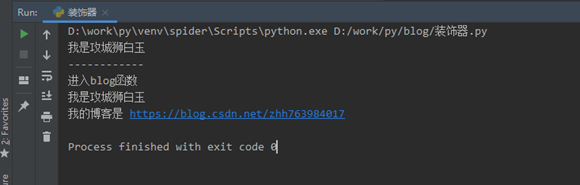接下来，我想知道这`baiyu``blog`两个函数分别的执行时间是多少，我就把代码修改如下：

``````import time

def baiyu():
t1 = time.time()
print("我是攻城狮白玉")
time.sleep(2)
print("执行时间为：", time.time() - t1)

def blog(name):
t1 = time.time()
print('进入blog函数')
name()
print('我的博客是 https://blog.csdn.net/zhh763984017')
print("执行时间为：", time.time() - t1)

if __name__ == '__main__':
func = baiyu  # 这里是把baiyu这个函数名赋值给变量func
func()  # 执行func函数
print('------------')
blog(baiyu)  # 把baiyu这个函数作为参数传递给blog函数``````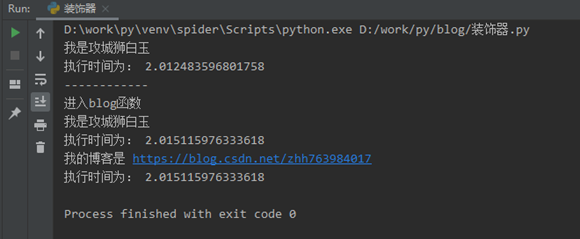上述的改写已经实现了我需要的功能，但是，当我有另外一个新的函数【python_blog_list】，具体如下：

``````def python_blog_list():
print('''【Python】爬虫实战，零基础初试爬虫下载图片（附源码和分析过程）
https://blog.csdn.net/zhh763984017/article/details/119063252 ''')
print('''【Python】除了多线程和多进程，你还要会协程
https://blog.csdn.net/zhh763984017/article/details/118958668 ''')
print('''【Python】爬虫提速小技巧，多线程与多进程（附源码示例）
https://blog.csdn.net/zhh763984017/article/details/118773313 ''')
print('''【Python】爬虫解析利器Xpath，由浅入深快速掌握（附源码例子）
https://blog.csdn.net/zhh763984017/article/details/118634945 ''')``````

``````def python_blog_list():
t1 = time.time()
print('''【Python】爬虫实战，零基础初试爬虫下载图片（附源码和分析过程）
https://blog.csdn.net/zhh763984017/article/details/119063252 ''')
print('''【Python】除了多线程和多进程，你还要会协程
https://blog.csdn.net/zhh763984017/article/details/118958668 ''')
print('''【Python】爬虫提速小技巧，多线程与多进程（附源码示例）
https://blog.csdn.net/zhh763984017/article/details/118773313 ''')
print('''【Python】爬虫解析利器Xpath，由浅入深快速掌握（附源码例子）
https://blog.csdn.net/zhh763984017/article/details/118634945 ''')
print("执行时间为：", time.time() - t1)``````# 三、简单的装饰器

``````import time

def baiyu():
print("我是攻城狮白玉")
time.sleep(2)

def count_time(func):
def wrapper():
t1 = time.time()
func()
print("执行时间为：", time.time() - t1)

return wrapper

if __name__ == '__main__':
baiyu = count_time(baiyu)  # 因为装饰器 count_time(baiyu) 返回的时函数对象 wrapper，这条语句相当于  baiyu = wrapper
baiyu()  # 执行baiyu()就相当于执行wrapper()``````

# 四、装饰器的语法糖@

baiyu = count_time(baiyu)

``````import time

def count_time(func):
def wrapper():
t1 = time.time()
func()
print("执行时间为：", time.time() - t1)

return wrapper

@count_time
def baiyu():
print("我是攻城狮白玉")
time.sleep(2)

if __name__ == '__main__':
# baiyu = count_time(baiyu)  # 因为装饰器 count_time(baiyu) 返回的时函数对象 wrapper，这条语句相当于  baiyu = wrapper
# baiyu()  # 执行baiyu()就相当于执行wrapper()

baiyu()  # 用语法糖之后，就可以直接调用该函数了``````

# 五、装饰器传参

``````def blog(name):
print('进入blog函数')
name()
print('我的博客是 https://blog.csdn.net/zhh763984017')``````

``````def count_time(func):
def wrapper(*args,**kwargs):
t1 = time.time()
func(*args,**kwargs)
print("执行时间为：", time.time() - t1)

return wrapper``````

``````import time

def count_time(func):
def wrapper(*args, **kwargs):
t1 = time.time()
func(*args, **kwargs)
print("执行时间为：", time.time() - t1)

return wrapper

@count_time
def blog(name):
print('进入blog函数')
name()
print('我的博客是 https://blog.csdn.net/zhh763984017')

if __name__ == '__main__':
# baiyu = count_time(baiyu)  # 因为装饰器 count_time(baiyu) 返回的时函数对象 wrapper，这条语句相当于  baiyu = wrapper
# baiyu()  # 执行baiyu()就相当于执行wrapper()

# baiyu()  # 用语法糖之后，就可以直接调用该函数了
blog(baiyu)``````

# 六、带参数的装饰器

``````import time

def count_time_args(msg=None):
def count_time(func):
def wrapper(*args, **kwargs):
t1 = time.time()
func(*args, **kwargs)
print(f"[{msg}]执行时间为：", time.time() - t1)

return wrapper

return count_time

@count_time_args(msg="baiyu")
def fun_one():
time.sleep(1)

@count_time_args(msg="zhh")
def fun_two():
time.sleep(1)

@count_time_args(msg="mylove")
def fun_three():
time.sleep(1)

if __name__ == '__main__':
fun_one()
fun_two()
fun_three()``````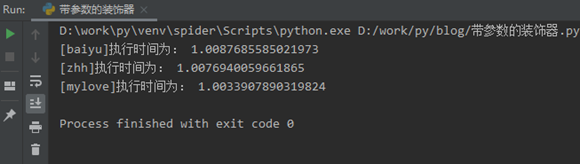# 七、类装饰器

• 通过__init__（）方法初始化类
• 通过__call__（）方法调用真正的装饰方法
``````import time

class BaiyuDecorator:
def __init__(self, func):
self.func = func
print("执行类的__init__方法")

def __call__(self, *args, **kwargs):
print('进入__call__函数')
t1 = time.time()
self.func(*args, **kwargs)
print("执行时间为：", time.time() - t1)

@BaiyuDecorator
def baiyu():
print("我是攻城狮白玉")
time.sleep(2)

def python_blog_list():
time.sleep(5)
print('''【Python】爬虫实战，零基础初试爬虫下载图片（附源码和分析过程）
https://blog.csdn.net/zhh763984017/article/details/119063252 ''')
print('''【Python】除了多线程和多进程，你还要会协程
https://blog.csdn.net/zhh763984017/article/details/118958668 ''')
print('''【Python】爬虫提速小技巧，多线程与多进程（附源码示例）
https://blog.csdn.net/zhh763984017/article/details/118773313 ''')
print('''【Python】爬虫解析利器Xpath，由浅入深快速掌握（附源码例子）
https://blog.csdn.net/zhh763984017/article/details/118634945 ''')

@BaiyuDecorator
def blog(name):
print('进入blog函数')
name()
print('我的博客是 https://blog.csdn.net/zhh763984017')

if __name__ == '__main__':
baiyu()
print('--------------')
blog(python_blog_list)``````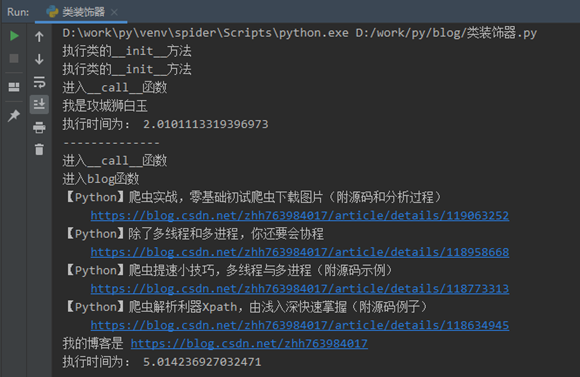# 八、带参数的类装饰器

``````class BaiyuDecorator:
def __init__(self, arg1, arg2):  # init()方法里面的参数都是装饰器的参数
print('执行类Decorator的__init__()方法')
self.arg1 = arg1
self.arg2 = arg2

def __call__(self, func):  # 因为装饰器带了参数，所以接收传入函数变量的位置是这里
print('执行类Decorator的__call__()方法')

def baiyu_warp(*args):  # 这里装饰器的函数名字可以随便命名，只要跟return的函数名相同即可
print('执行wrap()')
print('装饰器参数：', self.arg1, self.arg2)
print('执行' + func.__name__ + '()')
func(*args)
print(func.__name__ + '()执行完毕')

return baiyu_warp

@BaiyuDecorator('Hello', 'Baiyu')
def example(a1, a2, a3):
print('传入example()的参数：', a1, a2, a3)

if __name__ == '__main__':
print('准备调用example()')
example('Baiyu', 'Happy', 'Coder')
print('测试代码执行完毕')``````

# 九、装饰器的顺序

``````def BaiyuDecorator_1(func):
def wrapper(*args, **kwargs):
func(*args, **kwargs)
print('我是装饰器1')

return wrapper

def BaiyuDecorator_2(func):
def wrapper(*args, **kwargs):
func(*args, **kwargs)
print('我是装饰器2')

return wrapper

def BaiyuDecorator_3(func):
def wrapper(*args, **kwargs):
func(*args, **kwargs)
print('我是装饰器3')

return wrapper

@BaiyuDecorator_1
@BaiyuDecorator_2
@BaiyuDecorator_3
def baiyu():
print("我是攻城狮白玉")

if __name__ == '__main__':
baiyu()``````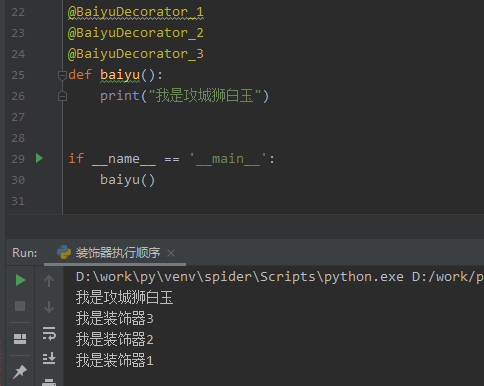``````@BaiyuDecorator_1
@BaiyuDecorator_2
@BaiyuDecorator_3
def baiyu():
print("我是攻城狮白玉")``````

baiyu = BaiyuDecorator_1 (BaiyuDecorator_2 (BaiyuDecorator_3(baiyu)))

我有话说: